# Bayesian Networks Bayesian networks A simple graphical notation

• Slides: 17Bayesian Networks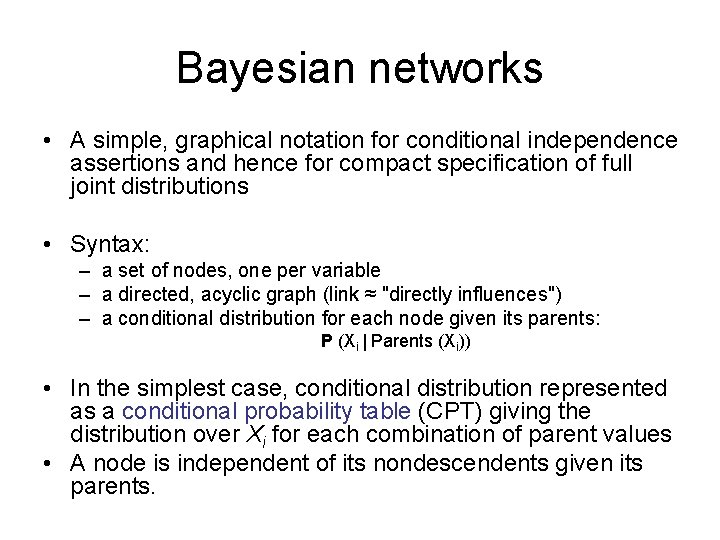Bayesian networks • A simple, graphical notation for conditional independence assertions and hence for compact specification of full joint distributions • Syntax: – a set of nodes, one per variable – a directed, acyclic graph (link ≈ "directly influences") – a conditional distribution for each node given its parents: P (Xi | Parents (Xi)) • In the simplest case, conditional distribution represented as a conditional probability table (CPT) giving the distribution over Xi for each combination of parent values • A node is independent of its nondescendents given its parents.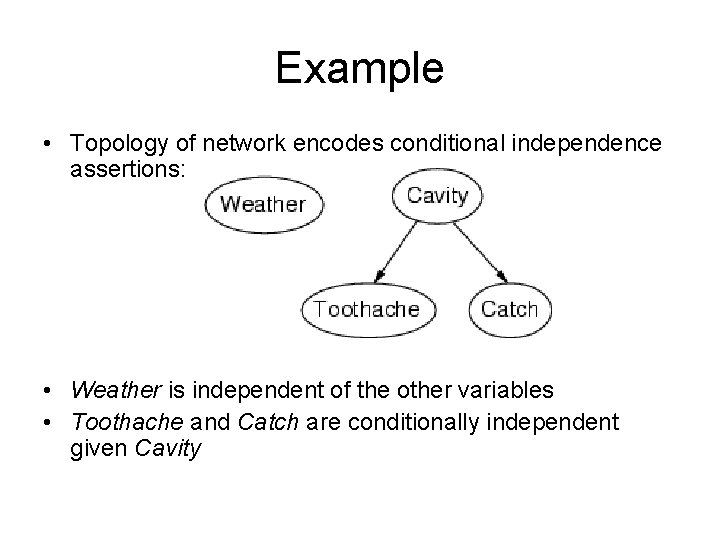Example • Topology of network encodes conditional independence assertions: • Weather is independent of the other variables • Toothache and Catch are conditionally independent given Cavity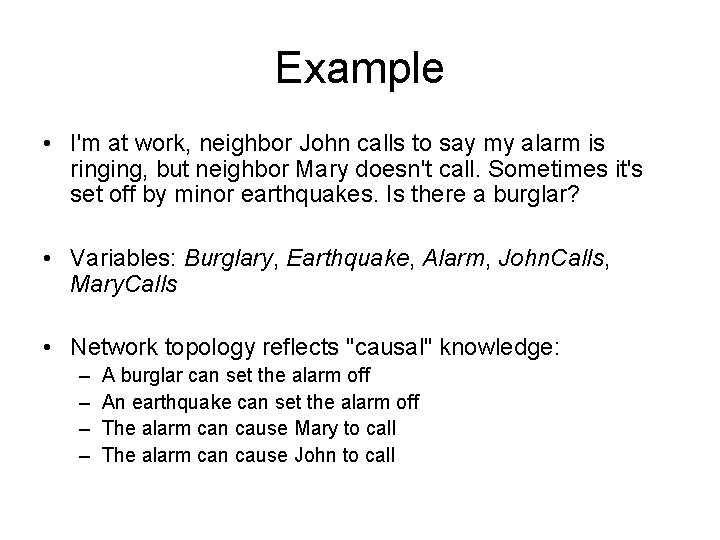Example • I'm at work, neighbor John calls to say my alarm is ringing, but neighbor Mary doesn't call. Sometimes it's set off by minor earthquakes. Is there a burglar? • Variables: Burglary, Earthquake, Alarm, John. Calls, Mary. Calls • Network topology reflects "causal" knowledge: – – A burglar can set the alarm off An earthquake can set the alarm off The alarm can cause Mary to call The alarm can cause John to callExample contd.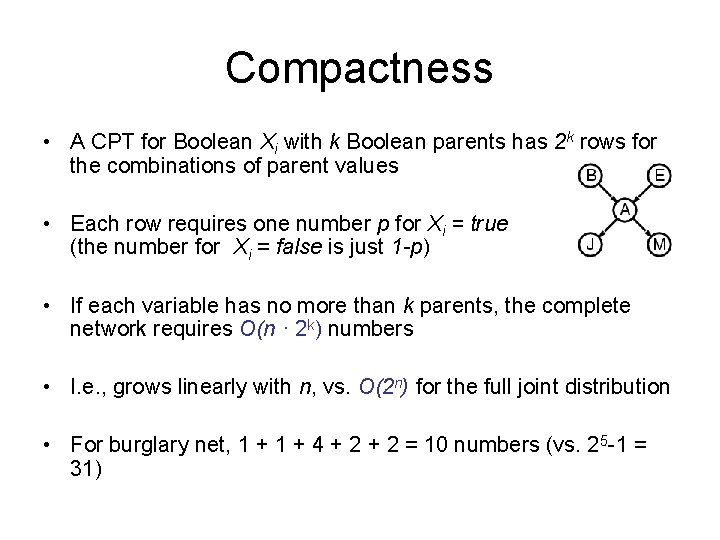Compactness • A CPT for Boolean Xi with k Boolean parents has 2 k rows for the combinations of parent values • Each row requires one number p for Xi = true (the number for Xi = false is just 1 -p) • If each variable has no more than k parents, the complete network requires O(n · 2 k) numbers • I. e. , grows linearly with n, vs. O(2 n) for the full joint distribution • For burglary net, 1 + 4 + 2 = 10 numbers (vs. 25 -1 = 31)Semantics The full joint distribution is defined as the product of the local conditional distributions: n P (X 1, … , Xn) = i = 1 P (Xi | Parents(Xi)) e. g. , P(j m a b e) = P (j | a) P (m | a) P (a | b, e) P ( b) P ( e) A node is independent of its non-descendents given its parents.Constructing Bayesian networks • 1. Choose an ordering of variables X 1, … , Xn • 2. For i = 1 to n – add Xi to the network – select parents from X 1, … , Xi-1 such that P (Xi | Parents(Xi)) = P (Xi | X 1, . . . Xi-1) This choice of parents guarantees: P (X 1, … , Xn) = πi =1 P (Xi | X 1, … , Xi-1) = πin=1 P (Xi | Parents(Xi)) n (chain rule) (by construction)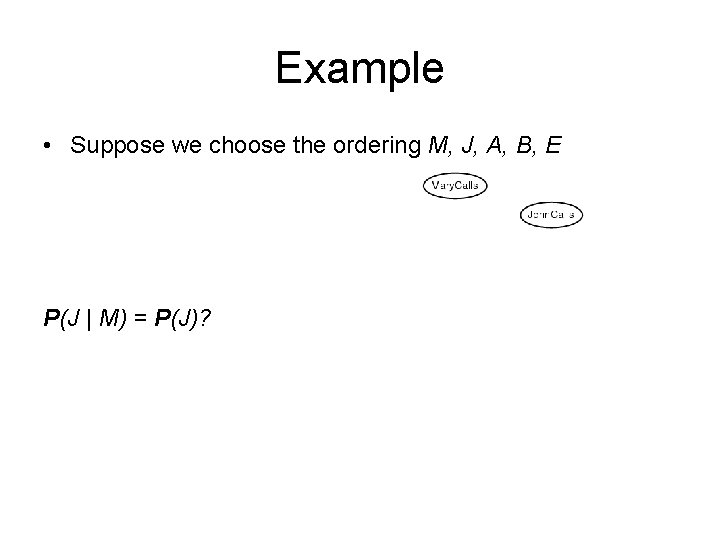Example • Suppose we choose the ordering M, J, A, B, E P(J | M) = P(J)?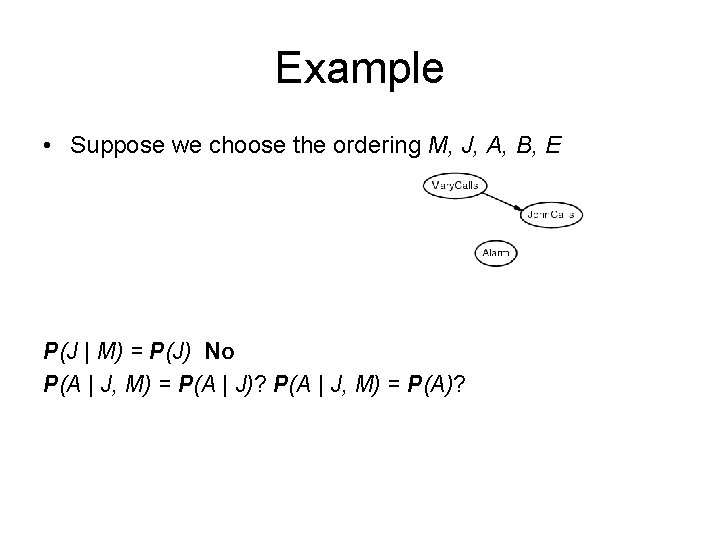Example • Suppose we choose the ordering M, J, A, B, E P(J | M) = P(J) No P(A | J, M) = P(A | J)? P(A | J, M) = P(A)?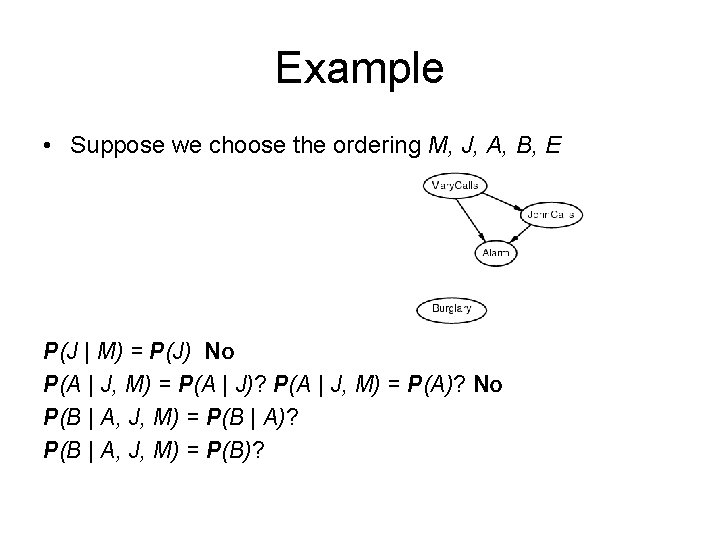Example • Suppose we choose the ordering M, J, A, B, E P(J | M) = P(J) No P(A | J, M) = P(A | J)? P(A | J, M) = P(A)? No P(B | A, J, M) = P(B | A)? P(B | A, J, M) = P(B)?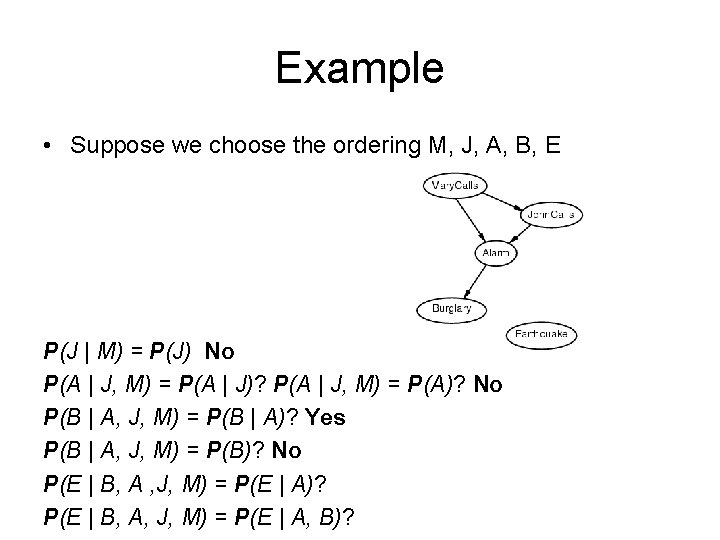Example • Suppose we choose the ordering M, J, A, B, E P(J | M) = P(J) No P(A | J, M) = P(A | J)? P(A | J, M) = P(A)? No P(B | A, J, M) = P(B | A)? Yes P(B | A, J, M) = P(B)? No P(E | B, A , J, M) = P(E | A)? P(E | B, A, J, M) = P(E | A, B)?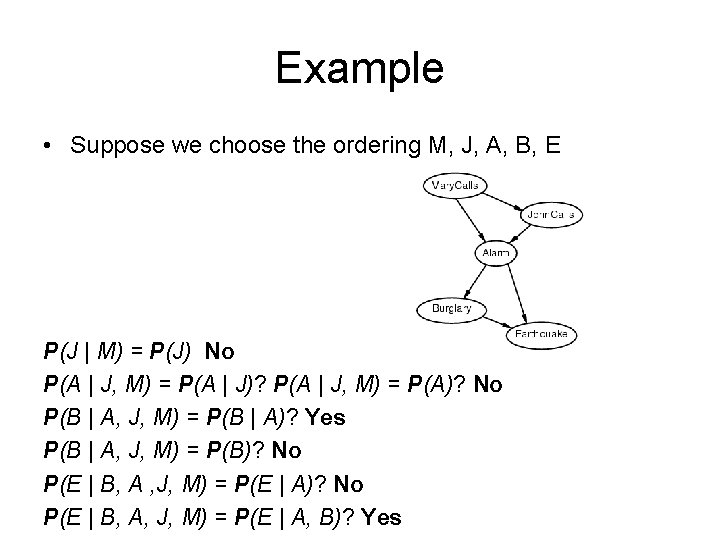Example • Suppose we choose the ordering M, J, A, B, E P(J | M) = P(J) No P(A | J, M) = P(A | J)? P(A | J, M) = P(A)? No P(B | A, J, M) = P(B | A)? Yes P(B | A, J, M) = P(B)? No P(E | B, A , J, M) = P(E | A)? No P(E | B, A, J, M) = P(E | A, B)? Yes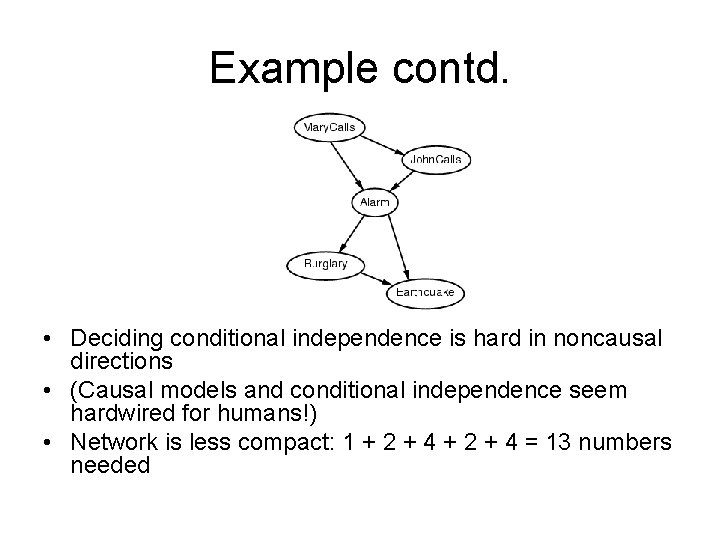Example contd. • Deciding conditional independence is hard in noncausal directions • (Causal models and conditional independence seem hardwired for humans!) • Network is less compact: 1 + 2 + 4 = 13 numbers neededConditional independence and D-separation • Two sets of nodes, X and Y, are conditionally independent given an evidence set of nodes, E if every undirected path from a node in X to a node in Y is d-seperated by E. • A set of nodes, E d-separates to sets of nodes, X and Y, if every undirected path from a node in X to a node in Y is blocked by E • A path is blocked given E if there is a node Z on the path for which one of the following holds: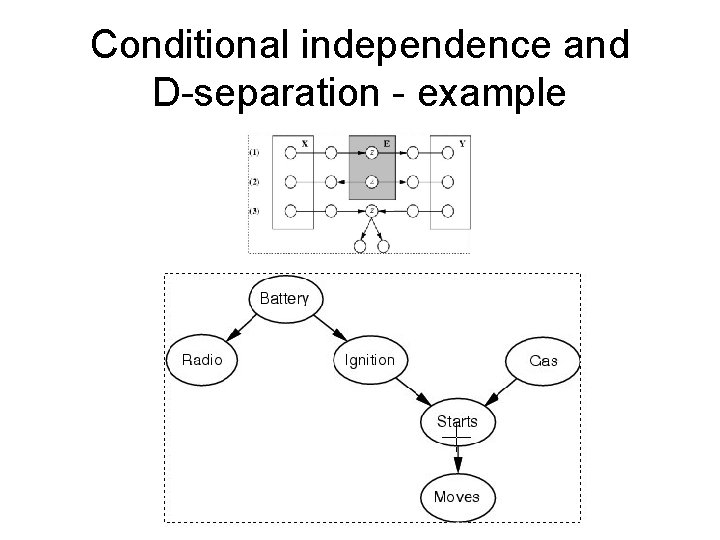Conditional independence and D-separation - exampleSome Applications of BN § Medical diagnosis § Troubleshooting of hardware/software systems § § Fraud/uncollectible debt detection Data mining Analysis of genetic sequences Data interpretation, computer vision, image understanding